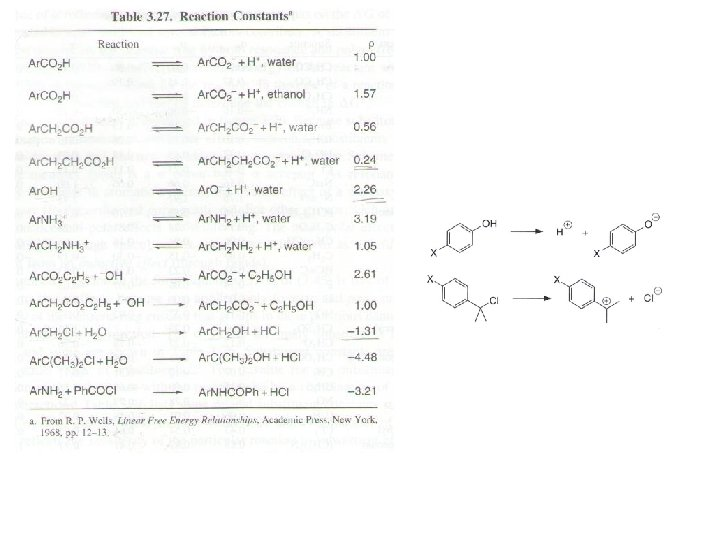# Hammett plots the most common linear free energy

• Slides: 19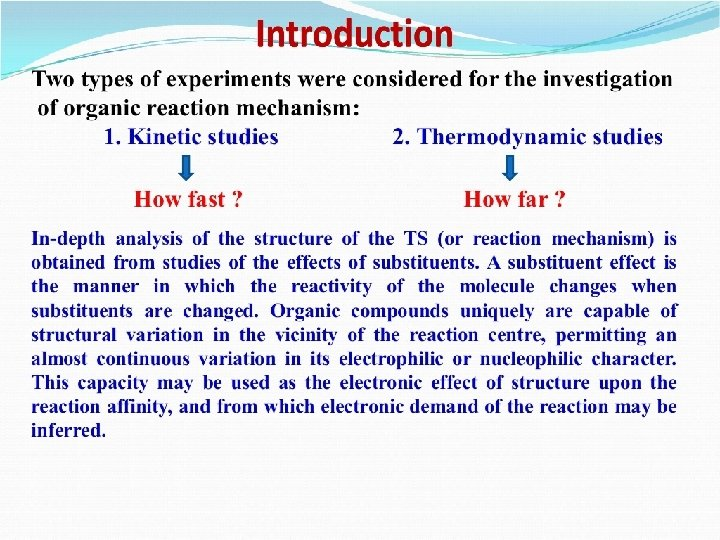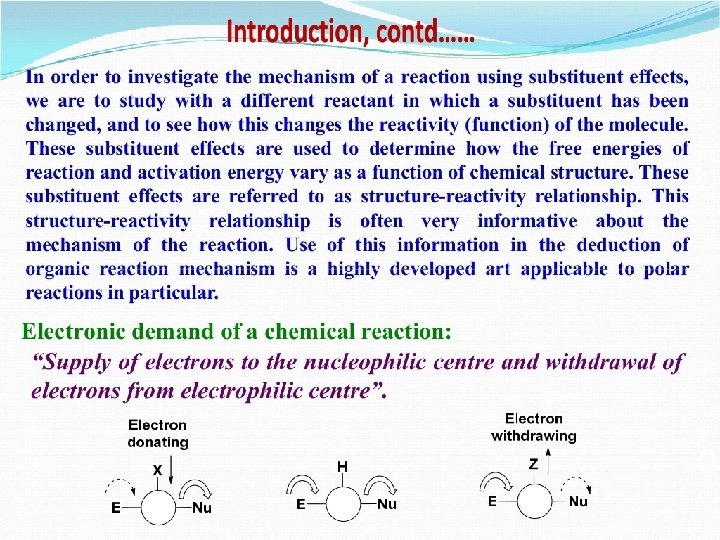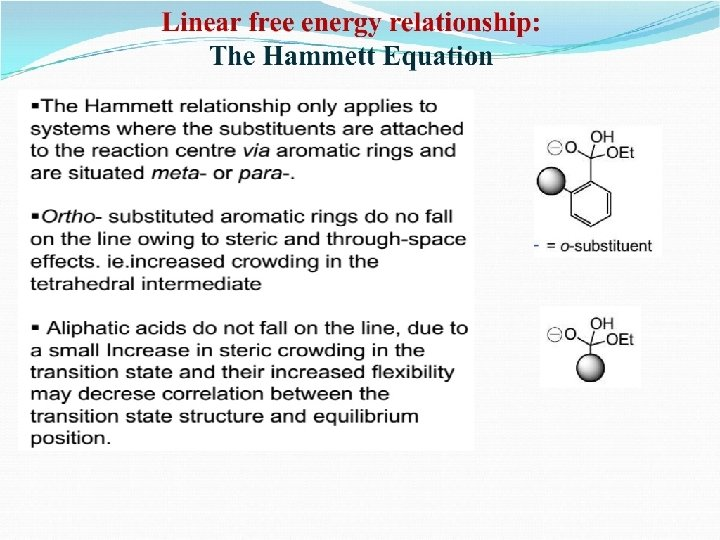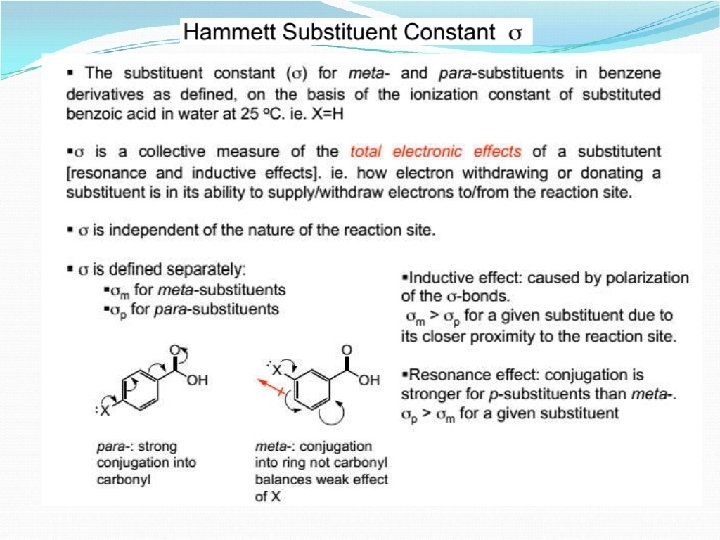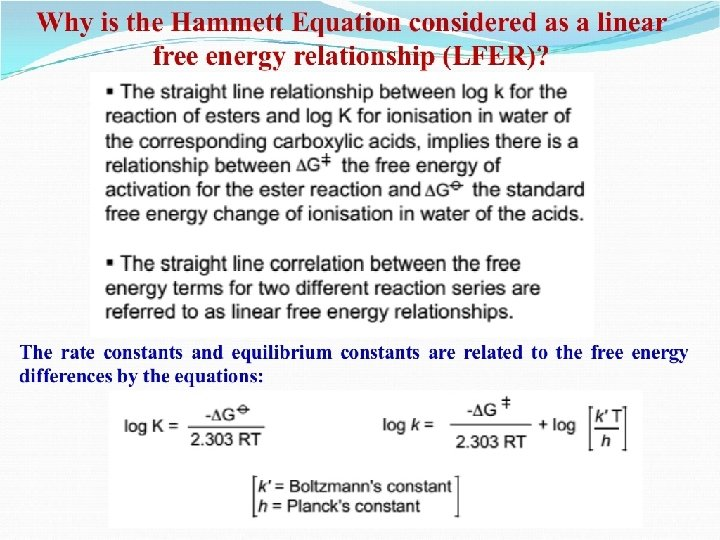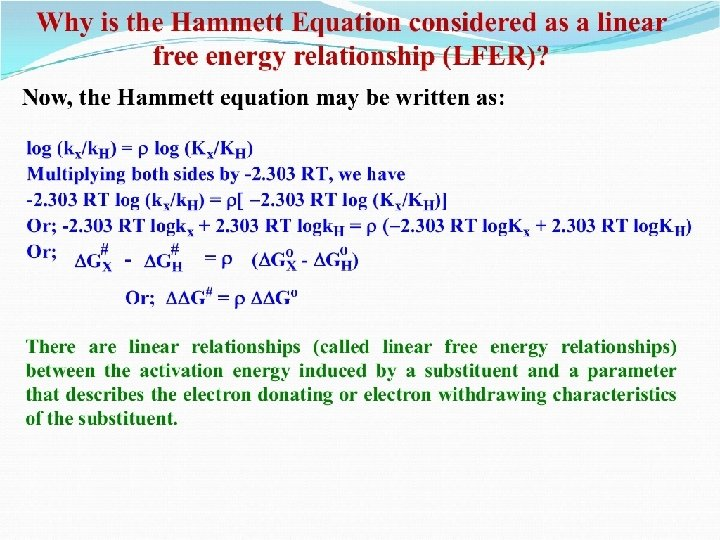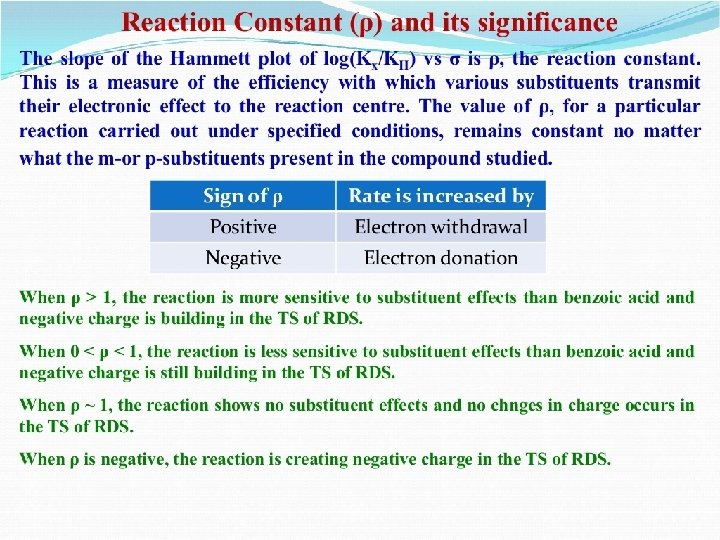Hammett plots – the most common linear free energy relationship. It is important to know effects of substituents on chemical properties. In particular, this contributes to understanding reaction mechanisms and to predicting rate constants and equilibrium constants. Hammett equation relates rates and equilibria for many reactions of compounds containing substituted phenyl groups. There is a relationship between the acid strengths of substituted benzoic acids and the rates of many other chemical reactions, for instance, the rates of hydrolysis of substituted ethyl benzoates. ko: rate constant for hydrolysis of ethyl benzoate k: rate constants for hydrolysis of substituted esters Ko: acid dissociation constant of benzoic acid (= Keq for X = H) K: acid dissociation constant of substituted acids (= Keq for X = substituents) log(k/ko) = mlog(K/Ko) DDG‡ = m. DDGo Linear free-energy relationship DGo = -RTlog. Keq k= kk. BT h e + + -DG /RT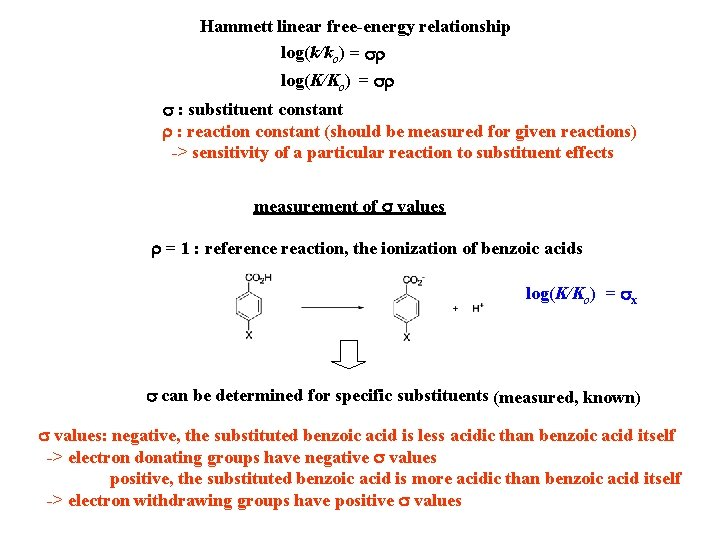Hammett linear free-energy relationship log(k/ko) = sr log(K/Ko) = sr s : substituent constant r : reaction constant (should be measured for given reactions) -> sensitivity of a particular reaction to substituent effects measurement of s values r = 1 : reference reaction, the ionization of benzoic acids log(K/Ko) = sx s can be determined for specific substituents (measured, known) s values: negative, the substituted benzoic acid is less acidic than benzoic acid itself -> electron donating groups have negative s values positive, the substituted benzoic acid is more acidic than benzoic acid itself -> electron withdrawing groups have positive s valuesinductive effect inductive and resonance effectss+ : used for reactions in which there is direct resonance interaction between an electron donor substituent and a cationic reaction center s- : used for reactions in which there is direct resonance interaction between an electron donor substituent and the electron-rich reaction site s+ s-Summary using Hammett equation for a particular reaction 1. Measure kx or Kx for substituents 2. Plot log(kx/ko) vs s 3. Determine r and interpret the results log(k/ko) = sxr log(K/Ko) = sxr log(k/ko) Slope = r s 1. When r > 1, the reaction under study is more sensitive to substituents than benzoic acid, and negative charge is building during the reaction. -> an electron withdrawing group stabilizes the developed negative charge. 2. When 0 < r < 1, the reaction is less sensitive to substituents than benzoic acid, but negative charge is still building. 3. When r is equal to or close to 0, the reaction shows no substituent effects. 4. When r is negative, the reaction is creating positive charge. -> electron donating groups increases in rates. 5. lrl large: much positive or negative charge separation